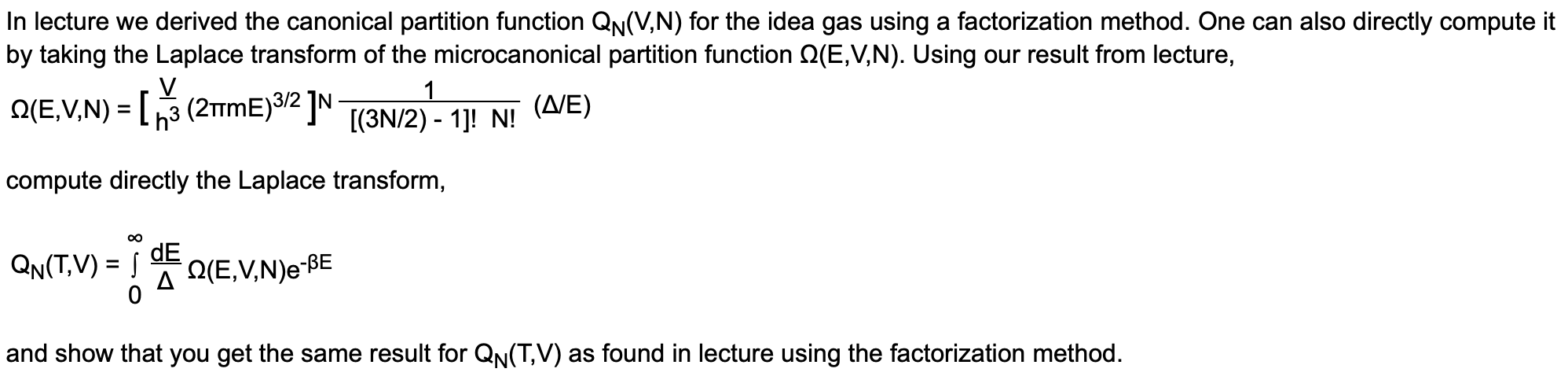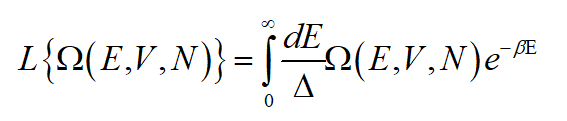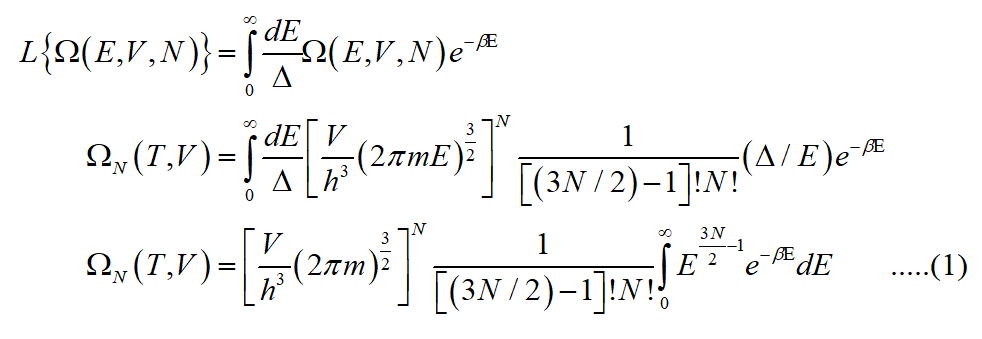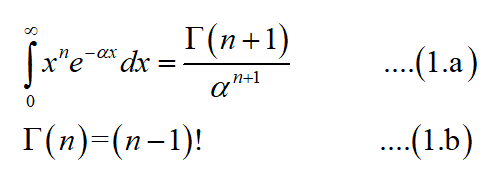# In lecture we derived the canonical partition function QN(V,N) for the idea gas using a factorization method. One can also directly compute itby taking the Laplace transform of the microcanonical partition function Q(E,V,N). Using our result from lecture,Q(E,V,N) = [3 (2TME)3/2 ]N[(3N/2) - 1]! N!(Δ/Ε)compute directly the Laplace transform,QN(T,V) = |dEA Q(E,V,N)e-BEand show that you get the same result for QN(T,V) as found in lecture using the factorization method.

Question
1 views

It's a statistical mechanics question.help_outlineImage TranscriptioncloseIn lecture we derived the canonical partition function QN(V,N) for the idea gas using a factorization method. One can also directly compute it by taking the Laplace transform of the microcanonical partition function Q(E,V,N). Using our result from lecture, Q(E,V,N) = [3 (2TME)3/2 ]N [(3N/2) - 1]! N! (Δ/Ε) compute directly the Laplace transform, QN(T,V) = | dE A Q(E,V,N)e-BE and show that you get the same result for QN(T,V) as found in lecture using the factorization method. fullscreen
check_circle

Step 1

Write the microcanonical partition function (E,V,N) and take the Laplace transform of it.Substitute the expression given for (E,V,N) in the above equation.Step 2

Use the gamma integral and gamma function property to calculate the above integral, i.e.,...

### Want to see the full answer?

See Solution

#### Want to see this answer and more?

Solutions are written by subject experts who are available 24/7. Questions are typically answered within 1 hour.*

See Solution
*Response times may vary by subject and question.
Tagged in